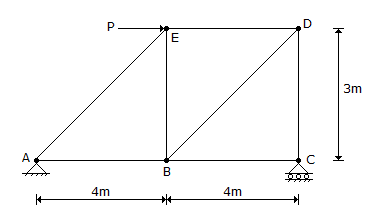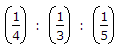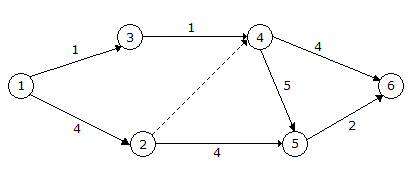# Civil Engineering - UPSC Civil Service Exam Questions

31.

What is the ratio of the forces in the members AB, BE and AE of the pin-joined truss shown in the figure?A. 5 : 4 : 3 B. 4 : 3 : 5 C.D. None of the above

Explanation:

No answer description available for this question. Let us discuss.

32.

A sewer, 1000 mm in diameter is laid at a slope to obtain a velocity of 0.8 m/s when running full. Assuming Manning's n as constant, what is the velocity in this sewer if it is running half full.

 A. 0.2 m/s B. 0.4 m/s C. 0.6 m/s D. 0.8 m/s

Explanation:

No answer description available for this question. Let us discuss.

33.

For the network shown in the above figure (the number on each arrow denotes the time duration of activity in days), the earliest star time, in days for activity 5-6 isA. 8 B. 7 C. 9 D. 11

Explanation:

No answer description available for this question. Let us discuss.

34.

A clay layer 5 m thick in field takes 300 days to attain 50% consolidation with condition of double drainage. If the same clay layer is underlain by hard rock then the time taken to attain 50% consolidation will be

 A. 300 days B. 600 days C. 900 days D. 1200 days

Explanation:

No answer description available for this question. Let us discuss.

35.

Two forces P = 6 N and Q = 10 N act on a particle and their lines of action are inclined to each other at an angle of 60°. What is the magnitude of the third force R which will keep the particle in equilibrium ?

 A. 13.30 N B. 13.89 N C. 14.00 N D. 14.02 N